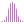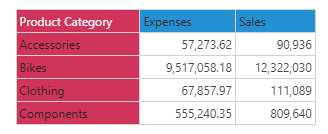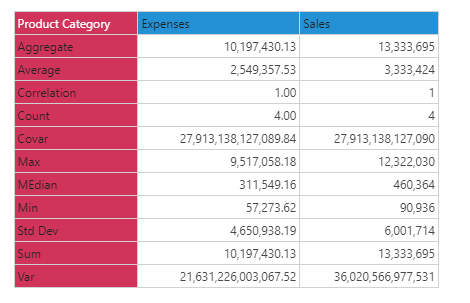#Count (PQL)

Returns the count of elements in the specified list.

#### Syntax

Count( <List> )

* Click on the function's arguments above for more details on the input values.

• List parameter should be a list or set of elements from an existing hierarchy in the model.
• For details on how to employ and use this function see the semantic calculation overview.
##### Different Function types
• This function is like the MDX count function; it calculates a count of elements in a list.
• If, instead, you wish to change the aggregate of a numeric column in the database, use this function.
• To use a simple count mathematical operation on a list of numbers, use the Common library function.

NOTE: There is no DISTINCT COUNT in PQL, as all counts in the semantic engine eliminate duplicates by default. To get a distinct count of elements use the granular function instead.

#### Examples

This example returns the count of product category elements in the product table/ dimension, using the sample demo model:

Count( {AllMembers([products].[Product Category])} )

Using the sample data below the count function would simply return 4: